## Surface Reconstruction

The grid-point-finding and color-matching algorithm supplies a list of screen coordinates, [a b], and their corresponding grid location [i j]. We would like to know the 3D point in the real world where each grid point has been projected. This amounts to solving two matrix equations: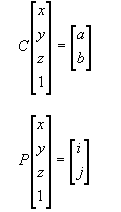...which can be combined into one block equation: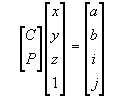To solve this, we split the C-P block matrix into the left 4x3 submatrix, which we'll call A, and the right 4x1 vector, which we'll call B. Rearranging the terms gives us: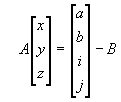Since this matrix is overdetermined, we solve using least squares.

The Matlab commands to do the calibration is located here. It prints out "uberMat" == (ATA)-1 * AT, the "B" vector, and some information about the projection. The least-squares solution can be calculated by: uberMat * ([a b i j]T - B).

In order to make a nice range image, we transform the resulting points to align them with the axes of the projector's space.

As it turns out, there are a lot of small holes in the range image, such as this one of Gary's face: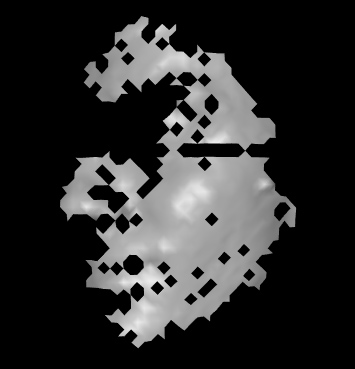In cases where one pixel is missing but the surrounding ones are there, we can easily interpolate the missing pixels. As long as there are no large depth discontinuities, we can interpolate even if there is just an upper and lower neighbor, or just a left and right neighbor (this kind of filling was not done for the Einstein bust, because it blurred over some discontinuities). Here is what the filled in face range image looks like: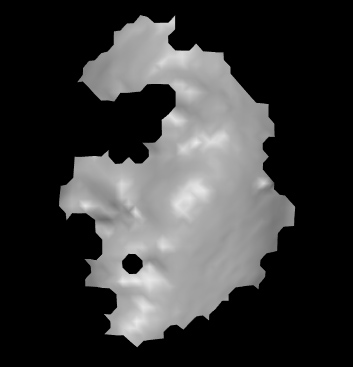Next, results...